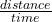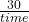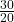## Find the Displacement A block slides 30 m at a constant velocity of 20 m/s. How much time did the trip take?

Question

Find the Displacement

A block slides 30 m at a constant velocity of 20 m/s.
How much time did the trip take?

in progress 0
5 months 2021-08-08T13:42:31+00:00 1 Answers 12 views 0

## Answers ( )

1. The trip time taken by the box is 1.5 sec.

Explanation:

Given:

distance(d) = 30 m

Velocity(v) = 20 m/s

By using formula:

Velocity =or, 20 =or, time(t) =∴ time (t) = 1.5 sec

Hence the the block took 1.5 sec to slide 30 m.